"Cloudster" Construction Project Session #43

Speed 400 Cloudster Project

Before starting work on recessing leading edge, it is instructive to measure and compare the "as built" overall vertical rise of each wing tip with the design value while the temporary leading edge is still in place.  The wing's inner panel end R1 rib replacements were glued in place, including the portion all the way forward to the temporary leading edge as shown below. Notice that the three turbulator spars are extend to these end R1 ribs as well and the trailing edge dihedral joint was reinforced with two larger 1/16" gussets (0.6" on a side) as shown below.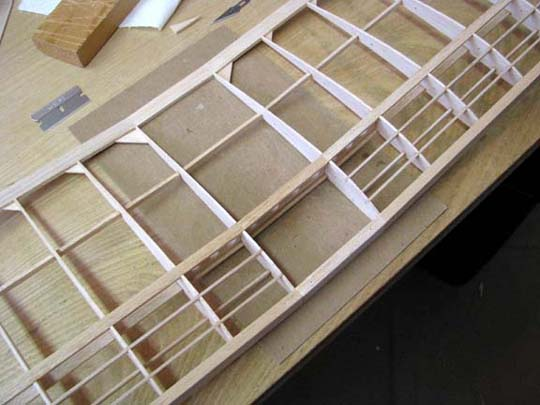The design value of the wing's vertical rise at the tip can be calculated from Report No. 33 as follows:
Design Vertical Rise = Hi' + Lt X Sin(J)
Design Vertical Rise = 1.257 + 12.027 X Sin(20.458) = 5.461"
Now to measure the vertical rise, the wing center section's leading and trailing edges were firmly weighted down to the work table with two square steel blocks as shown below. Then a triangular scale also shown below was used to measure the wing tip vertical rise off of the work table.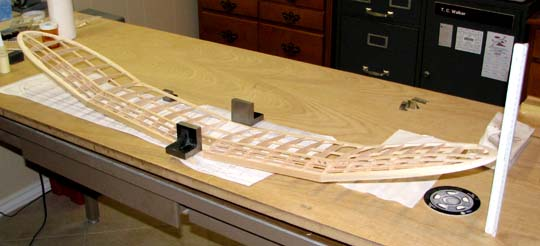The vertical rise measurement of the lower edge of the left wing tip off the work table, as viewed from the front leading edge, is 4-15/16" as seen below.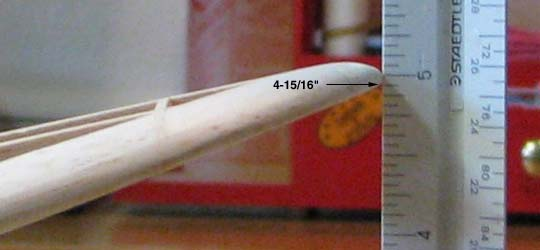This however is not the true measurement because there is a 7/16" (0.438") blank section at the end of the scale as shown below. The true measurement is (4.938+0.438) = 5.376". Therefore, left wing tip rise is slightly under the design value by 5.376-5.461 = -0.085", which is only a 1.6% overall error.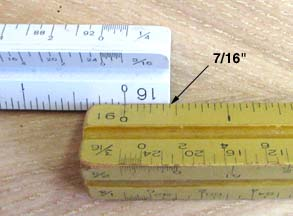The vertical rise measurement of the lower edge of the right wing tip off the work table, as viewed from the trailing leading edge, is 5" as seen below, which is 5+0.438 = 5.438". The right wing tip rise is slightly under the design value by 5.438-5.461 = -0.023", which is only a 0.4% overall error.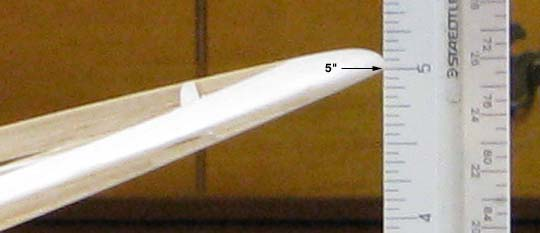These errors are well within practical building tolerances for balsa. I am pleased and probably very lucky that these errors turned out to be so small........................Tandy